Sine Function Transformations

In this page we use geogebra (or another graphing software) to help understand transformations of sine functions.

On the first task, four interactive geogebra pages have been prepared to introduce the concepts.

The next two tasks are simply to get you going using geogebra (or other) as a learning tool.

Dynamic Worksheets on Geogebra: explore the sine curve. You do not need a geogebra account – if you do not wish to have your answers stored, simply press cancel when prompted to make an account.

This video will guide you through the steps required to draw the following four graphs on geogebra.

Using the guidance on the video (if required), draw these four graphs on geogebra.

Project 1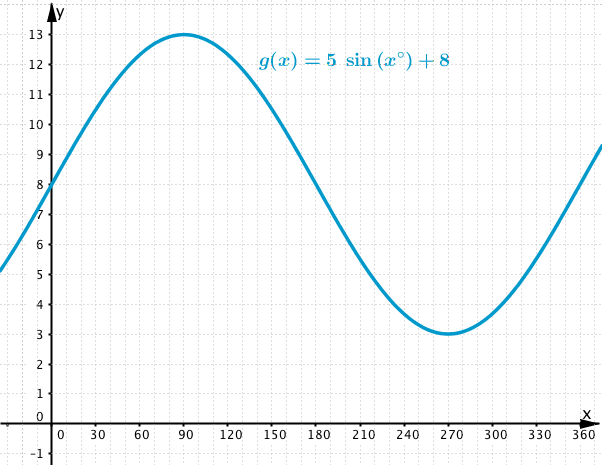Project 2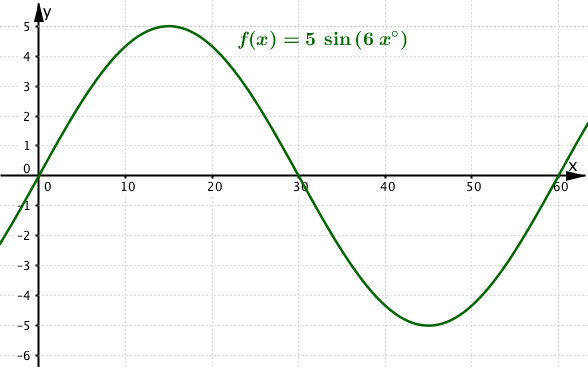Project 3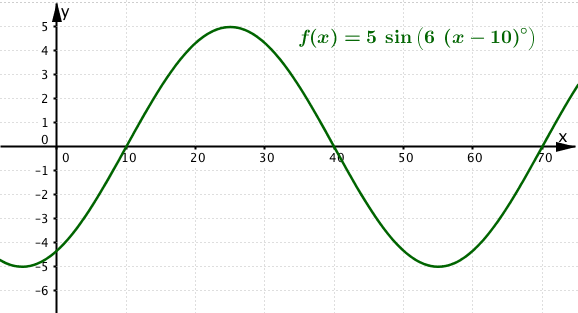Project 4Using your knowledge of transformations of circular functions, figure out a correct equation for each of the problems found below. For a printable copy click Sine Function Transformations Problems 1 – 4. You may use your own geogebra, the applet directly below the problems or of course, your preferred graphing calculator. Whichever method, match your graph to the one given. When you have the correct graph, click on show solutions at the end of the page to check your equation. Working in pairs is recommended!

Problem 1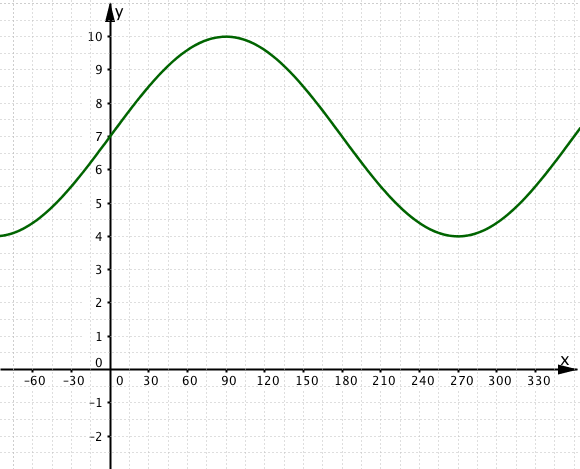Problem 2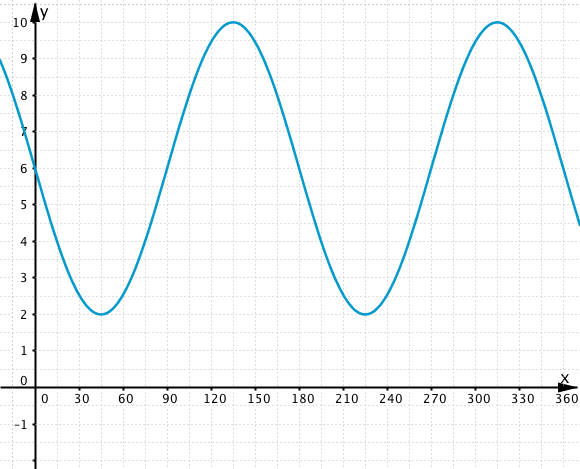Problem 3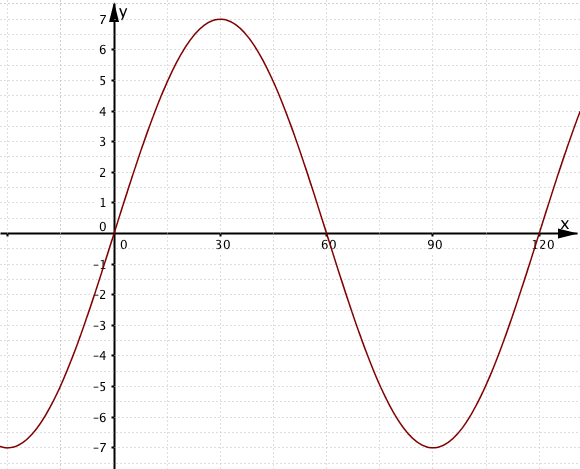Problem 4